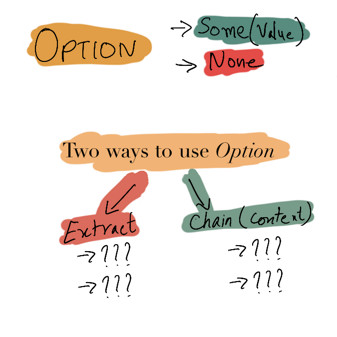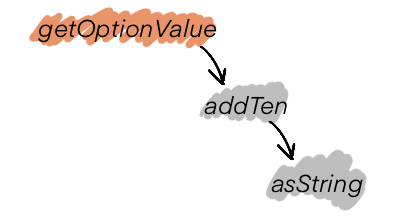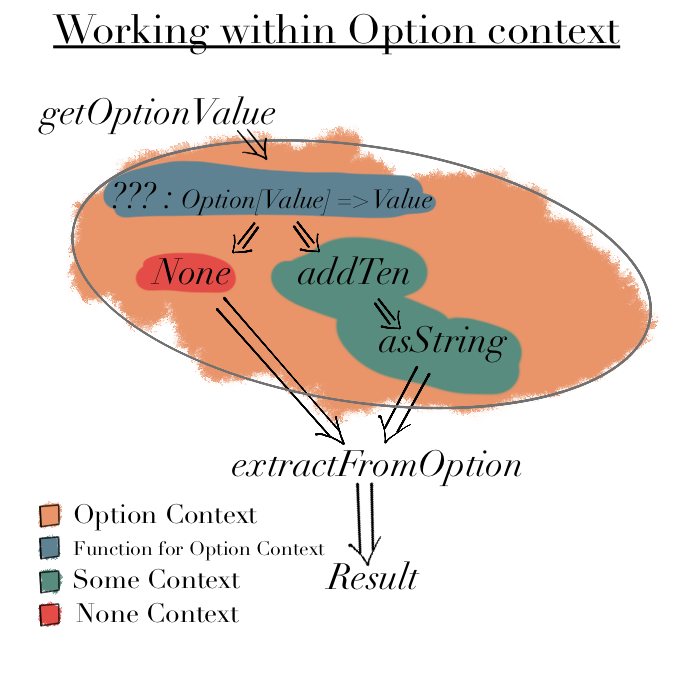# FP for Sceptics: Introduction to Option Type

Functional Programming (FP) is based around mathematical concepts like Type Theory - We define our system in terms of ADTs, data flow & functions1.

We first implement "Happy Path" and then implement handlers for "Unhappy Path" (error handling). In `ADTs in Practice` we used "Exceptions" (`IO.raiseError`) for error handling.

However FP promotes using types for error handling, such as:

• `Option`
• `Either`
• `Monad`
• etc.

In this post we will start by looking at the simplest of these:

`Option` type denotes presence (`Some(value)`) or absence (`None`) of a value.In programming, we use functions and methods to do any action and return a value2. Depending upon the logic we may or may not have a return value.

Imperative Programming Languages (Java, Python etc) express this using either `null` or an `Exception`.

In Functional programming we can use `Option` type to say the value `may exist`.

Is this practical? Consider retrieving a value from a HashMap or a Dictionary.

```val hMap: Map[Int, Int] = Map(1 -> 102, 2 -> 202, 3 -> 302)

hMap.get(100)
// What should be the value here?
// `100` does not exist in `hMap`
```

In non-FP languages, we need to do null check or have exception handling around `hMap.get`.

In FP languages, `hMap.get` will return an `Option` and depending upon the flow of the program, we have two ways of dealing with it:

• Extract value
• Work within Option context

## Extract value

This is useful when we want to directly work with a value, whether it exists or not.

There are two ways to extract a value from an `Option`

• getOrElse
• Pattern Matching

### `getOrElse`

This is idiomatic in Scala and similar function/method is available in other imperative languages as well.

```def getFromOption(opt: Option[String]): String =
"Got Value: " + opt.getOrElse("None")

getFromOption(Some("100")) // "Got Value: 100"

getFromOption(None) // "Got Value: None"
```

### Pattern Matching

Pattern Matching is the "functional way" of extracting a value.3

```def extractFromOption(opt: Option[String]): String =
opt match {
case None        => "Got Value: " + "None"
case Some(value) => "Got Value: " + value
}

extractFromOption(Some("100")) // "Got Value: 100"

extractFromOption(None) // "Got Value: None"
```

### What about `.get`?

`Option` type also has `.get` method but it is unsafe to use as `None.get` will throw an exception and we want to avoid running into such errors.

Note: `Option` ensures that we will never run into `NullPointerException` (NPE) thanks to `.getOrElse` & pattern matching4.

## Work within Option context

This is useful when we want to work with an `Option` type without extracting the value at every turn, which will get cumbersome.

Consider the flow:which is defined by the following functions

```val hMap: Map[Int, Value] =
Map(
1 -> Value(102),
2 -> Value(202),
3 -> Value(302)
)

def getOptionValue(i: Int): Option[Value] = hMap.get(i)

def addTen(i: Value): Value10 = Value10(i.value + 10)

def asString(num: Value10): String = s"\${num.value}"

case class Value(value: Int) extends AnyVal
case class Value10(value: Int) extends AnyVal
```

There are two ways of working within `Option context`### Using `map` to compose our functions

`map` is useful when a function in the chain returns an `Option` and rest of functions need to be executed only if function's input is available.

```def mapOpt(i: Int): Option[String] =
getOptionValue(i)
.map(asString)

extractFromOption(mapOpt(3)) // "Got Value: 312"

extractFromOption(mapOpt(100)) // "Got Value: None"

def extractFromOption(opt: Option[String]): String =
opt match {
case None        => "Got Value: " + "None"
case Some(value) => "Got Value: " + value
}
```

### Using `for-comprehension` or `flatMap`5

`for-comprehension` (and `flatMap`) are useful when multiple functions in the chain return `Option` and but we only want to execute the next function if function's input is available.

```// Using for-comprehension
def forOpt(i: Int): Option[String] =
for {
value <- hMap.get(i)
str   <- Option.apply(asString(val10))
} yield str

// Using flatMap
def flatMapOpt(i: Int): Option[String] =
getOptionValue(i)
.flatMap(val10 => Option.apply(asString(val10)))

// for-comprehension
extractFromOption(forOpt(3)) // "Got Value: 312"
extractFromOption(forOpt(100)) // "Got Value: None"

// flatmap
extractFromOption(flatMapOpt(3)) // "Got Value: 312"
extractFromOption(flatMapOpt(100)) // "Got Value: None"

def extractFromOption(opt: Option[String]): String =
opt match {
case None        => "Got Value: " + "None"
case Some(value) => "Got Value: " + value
}
```

Note: The code is a straightforward to read because we need not check for `None` at every step.

## Conclusion

In this post we looked at `Option` type - what, how & why to use it.

We saw how it:

• Elegantly handles possible `null` cases so that we don't run into `NullPointerException` or similar issues.
• Makes code declarative/functional to read.

In my next post I will cover where, when and to what extent `Option` type is useful in practical applications.

Would you like a free ebooklet?

#### Code

Complete code can be found at: Gist 9554bf80cf98aede3304faa190db2938

1. For sake of simplicity we are ignoring mutablity and impure functions.

2. Pattern Matching is quite versatile as we will see in case of `Either`. I plan on writing a separate post about its versatility.

3. The caveat is that if we have `Option[None]` then we risk NPE at next level. However `Option[None]` is a code smell and needs to be fixed.

4. If you are unfamiliar with how `flatMap` or `for-comprehension` works, you can ignore `for-comprehension` for now. I will be covering `map/flatMap` in a future post.